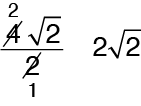## 1.2 Learn Basic Properties and Operations

This lesson will help you learn the basic properties and rules that govern math problem-solving.

When a radical is in the denominator, we usually use multiplication to remove the radical. We will use the idea that for any number, the square of a square root equals the number itself: $$\sqrt a \,\cdot \,\sqrt a \, = a$$.
Simplify $${\textstyle{4 \over {\sqrt 2 }}}$$.
 $${\textstyle{4 \over {\sqrt 2 }}}\,\,\cdot \,{\textstyle{{\sqrt 2 } \over {\sqrt 2 }}} = {\textstyle{{4\sqrt 2 } \over 2}}$$ We want to remove the radical from the denominator. We can multiply the fraction by $${\textstyle{{\sqrt 2 } \over {\sqrt 2 }}}$$ without changing the value of the fraction.We can divide both the top and bottom by 2. In simplest form, $${\textstyle{4 \over {\sqrt 2 }}} = 2\sqrt 2$$.